## Solutions Homework Set # 1 - Physics 122

Problem 1.

Since the two spheres are identical, the total charge will be distributed equally across them when they touch. The total initial charge is equal to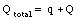The final charge is thus equal to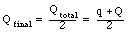Problem 2.

The electric field at the midpoint due to charge Q is equal to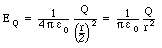The electric field at the midpoint due to charge q is equal to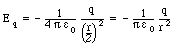The minus sign in this equations indicates that for a positive charge q, the electric field at the midpoint will point towards the left. The total electric field at the midpoint is the vector sum of the electric fields generated by the two charges: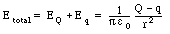The direction of the electric field at the midpoint is either to the left or to the right, depending on which of the two charges is more positive. Note: the problems asks for the magnitude of the electric field!

Problem 3.

The electric force between the charges in the final configuration is equal to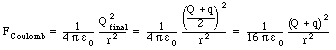Problem 4.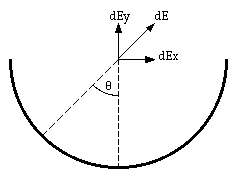Figure 1. Problem 4.

On the basis of the symmetry of the charge distribution we can conclude that the electric field at P will either be pointing straight up or straight down, for positive and negative charge distributions, respectively. Consider a small segment of the plastic rod at angle [theta]. If the angular width of this segment is equal to d[theta], then the amount of charge on this segment is equal to: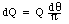The magnitude of the total electric field at P due to this small segment is equal to: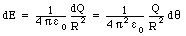Because of the symmetry argument, we only need to consider the vertical component of this field: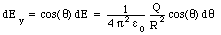In order to obtain the total electric field at P we need to sum over all segments, which is equivalent to varying [theta] between -[pi]/2 and +[pi]/2: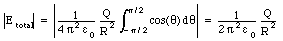Problem 5.

When the balls are hanging vertically, there are only two forces acting on them: the gravitational force and the tension. These are acting in opposite directions, and since the system is at rest, the net force acting on the balls must be equal to 0 N. Thus: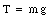Note: make sure you user the correct units. If you want to calculate the tension in Newton, you need to use kg for the mass.

Problem 6.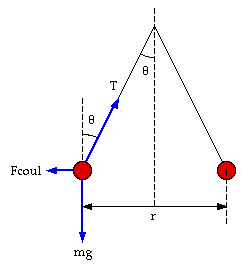Figure 2. Problem 6: forces on ball.

After charge is added to the system, there are three forces acting on each ball: the gravitational force, the tension, and the electric force. These forces are shown schematically in Figure 2. Since the system is at rest, the net force on each ball must be equal to zero. Consider the vertical component of the net force acting on each ball: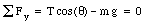This equation tells us immediately what the tension in each thread is: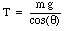Problem 7.

Consider the horizontal component of the net force acting on each ball: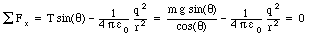Here, r is the distance between the two charged balls. This equation can be rewritten to solve for r: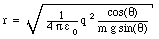Using trigonometry we can determine the following relation between the angle [theta], the length of the string l, and the distance r:This equation can be used to solve for l: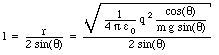Problem 8.

Electric breakdown occurs when the electric field reaches a critical value Ecrit. The force on a free electron at this field is equal to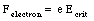The resulting acceleration is equal to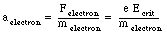Assuming the electron starts from rest, its velocity at time t will be equal to: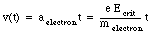Its kinetic energy at time t will be equal toThe problem states the kinetic energy of the electron. The previous equation can be used to determine at what time the electron achieves this kinetic energy: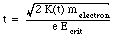At that time, the electron will have moved a distance d equal to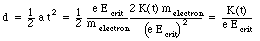Problem 9.

Use the graph shown on your assignment to determine the electric field at the location of interest E(x). The force on a particle of charge q is equal to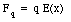The corresponding acceleration experienced by this particle is thus equal to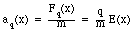Problem 10.Figure 3. Problem 10.

To calculate the electric field generated by the rectangular charge distribution we first consider the electric field generated by one side, for example the side of length L. The derivation of the electric field is very similar to the derivation of the electric force on a point charge q due to an infinitely long straight charge distribution (see Example 2 in Chapter 23), except that instead of integrating over [theta] between -[pi]/2 and [pi]/2, we need to integrate over a smaller angular range.

Consider the situation shown in Figure 3. The maximum angle [theta] is equal to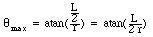The electric field at the position indicated in Figure 3 can now be calculated from eq.(12) in Chapter 23 by changing the integration limits: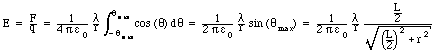In the problem we have two rods of length L separated by a distance W. The electric field at A will be pointing in the vertical direction since the horizontal components cancel (see Figure 4). The previous equation can be used to calculate the magnitude of the electric field due to these two charge distributions: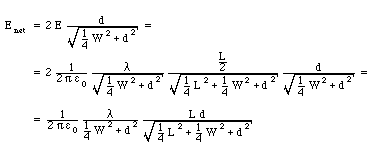A similar formula can be derived the field at A generated by the two rods of length W. The total electric field at A is equal to the sum of these fields: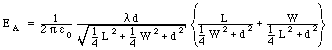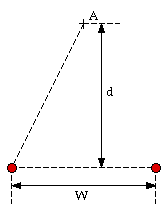Figure 4. Problem 10.